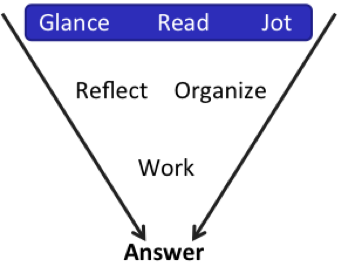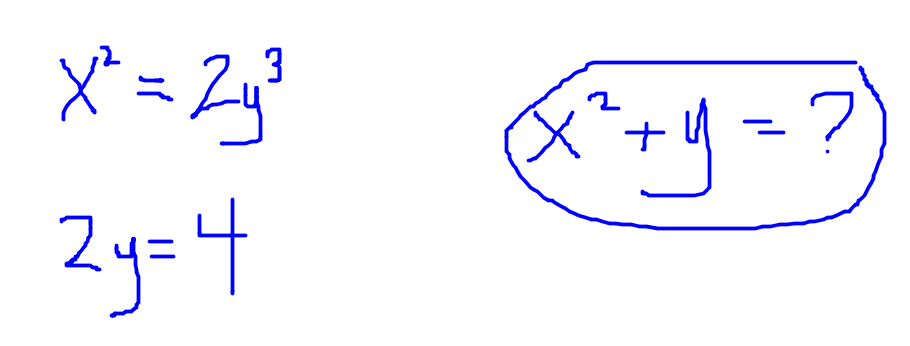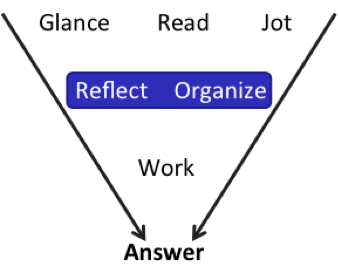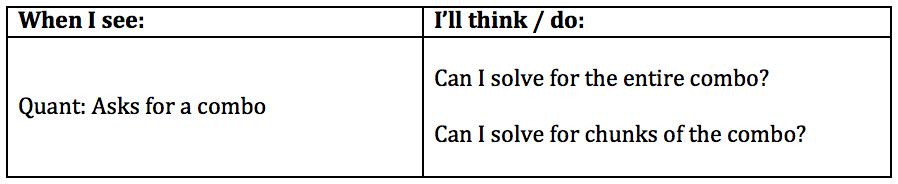# Know the GMAT Code: Working Backwards - Part 2

by , Jul 29, 2017Last time, I asked whether you know howand whento Work Backwards on Problem Solving (PS) problems. If you havent already worked through part 1 of this series, go do that and then come back here. Ill wait. :)

Heres the second GMATPrep problem from that set:

"If [pmath]x^2= 2y^3[/pmath]and [pmath]2y = 4[/pmath], what is the value of [pmath]x^2+ y[/pmath]?

(A) -14

(B) -2

(C) 3

(D) 6

(E) 18

Lets discuss!At first glance, this one does look like it might be a Working Backwards problem: The answers are pretty nice integers.

But! Whats the issue? Why wouldn't we actually want to work backwards on this one?

Look at the question stem:The strategy works really well when the question asks you for one discrete variablenot when it asks you for a combo (combination of variables), as in this problem.

Why? Try it out. Lets say that [pmath]x^2+ y = 6[/pmath] (answer D). What are the values for [pmath]x[/pmath]and [pmath]y[/pmath]individually? Or even [pmath]x^2[/pmath]and [pmath]y[/pmath] individually?

There are a lot of possibilities. I dont want to try a lot of possibilities. Im lazy; I want to get this problem done as easily as possible! So working backwards isnt a good approach on this one.What should I do instead? One of the equations is super easy: [pmath]2y = 4[/pmath]. Now I know that [pmath]y = 2[/pmath], and the problem is a whole lot easier to approach.

Plug [pmath]y = 2[/pmath] into the other equation and wait.

Reflect a little more. What else do you need to know in order to answer the question?

[pmath]x^2[/pmath]. Not [pmath]{x!}[/pmath] So dont solve for [pmath]x[/pmath]; instead, solve for [pmath]x^2[/pmath]. Dont do more work than you have to do.

[pmath]x^2= 2y^3[/pmath]

[pmath]x^2= 2(2)^3[/pmath]

[pmath]x^2= 2(8)[/pmath]

[pmath]x^2= 16[/pmath]

Finally, find the value of [pmath]x^2+ y[/pmath].

[pmath]x^2+ y[/pmath]

[pmath]16 + 2 = 18[/pmath]

One more question: Where do you think the wrong answers came from? Theyre not randomthey were chosen because they represent some kind of specific mistake that people are likely to make. If you can spot how the wrong answer was created, you are arming yourself against making those kinds of mistakes yourself.

In this case, lets say that you did solve all the way for x. And then maybe you forget that they wanted [pmath]x^2+ y[/pmath]so you solve just for [pmath]x + y[/pmath]. Thats 6, or trap answer (D).

And if you do solve for [pmath]x[/pmath], then youd actually get [pmath]x = 4[/pmath] and 4. And 4 + 2 = 2, aka trap answer (B).

Trap answer (A) appears to come from adding 16 and 2, so that would be a couple of mistakes (because when you square 4, you should get a positive value, not a negative one).

I havent figured out where trap answer (C), 3, comes from. If youve got any ideas, share them in the comments.

The big takeaway for me on these wrong answers: Multiple of them revolve around solving all the way for [pmath]x[/pmath]rather than solving for [pmath]x^2[/pmath]. In other words, besides saving time, Im also saving myself from making careless mistakes when I pause and make sure that Im solving only for exactly what I need on this GMAT problem, not for what a math teacher would make me do.

## Key Takeaways for Knowing the Code:

(1) If the question asks for a combo (combination of variables), then working backwards probably wont be a good strategy. Look for another angle.

(2) When a question does ask for a combo, think about how to solve directly for the entire combo or, at the least, for chunks of the combo. Dont just auto-solve for all of the individual variables, the way you were taught in school.

(3) Turn that knowledge into Know the Code flash cards:* GMATPrep questions courtesy of the Graduate Management Admissions Council. Usage of this question does not imply endorsement by GMAC.